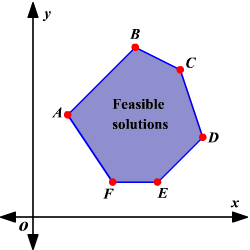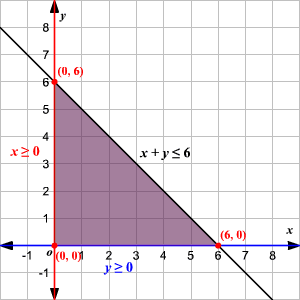# Linear Programming

A linear programming problem may be defined as the problem of maximizing or minimizing a linear function subject to system of linear constraints. The constraints may be equalities or inequalities. The linear function is called the objective function , of the form $f\left(x,y\right)=ax+by+c$ . The solution set of the system of inequalities is the set of possible or feasible solution , which are of the form $\left(x,y\right)$ .

If a linear programming problem can be optimized, an optimal value will occur at one of the vertices of the region representing the set of feasible solutions.For example, the maximum or minimum value of $f\left(x,y\right)=ax+by+c$ over the set of feasible solutions graphed occurs at point $A,B,C,D,E$ or $F$ .

When the graph of a system of inequalities forms a region that is closed, the region is said to be bounded. Sometimes a system of inequalities forms a region that is open. In this case, the region is called unbounded.

To solve a linear programming problem, follow these steps.

$•$ Graph the region corresponding to the solution of the system of constraints.

$•$ Find the coordinates of the vertices of the region formed.

$•$ Evaluate the objective function at each vertex to determine which $x$ - and $y$ -values, if any, maximize or minimize the function.

Example:

Find the minimum value and maximum value of the objective function $f\left(x,y\right)=4x+5y$ , subject to the following constraints.

$\left\{\begin{array}{l}x\ge 0\\ y\ge 0\\ x+y\le 6\end{array}$

Solution

First graph the region corresponding to the solution of the system of constraints.Now find the coordinates of the vertices of the region formed.

The vertices are $\left(0,0\right)$ , $\left(0,6\right)$ , and $\left(6,0\right)$ .

Evaluate the objective function at each vertex.

At $\left(0,0\right):f\left(0,0\right)=4\left(0\right)+5\left(0\right)=0$ , Minimum value of $f\left(x,y\right)$

At $\left(0,6\right):f\left(0,6\right)=4\left(0\right)+5\left(6\right)=30$ , Maximum value of $f\left(x,y\right)$

At $\left(6,0\right):f\left(6,0\right)=4\left(6\right)+5\left(0\right)=24$

So, the maximum value of $f$ is $30$ when $x=0$ and $y=6$ . The minimum value of $f$ is $0$ when $x=0$ and $y=0$ .